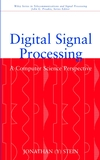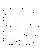# Errata - all known errors

updated February 2005

page line incorrect correct
18 equation (2.2)
 lim N -> 0
remove limit
27 17 sin(w1t) cos(w2t) + cos(w1t) sin(w2t) 1/2( cos((w1-w2)t) - cos((w1+w2)t) )
28 table - line 7 an A an
29 exercise 2.2.7 1 / SQRT T 1 / T
30 exercise 2.2.9 d(t) d(x)
32 1 When measuring in, When measuring in dB,
33 2 n=-∞,∞ n=-∞ ... ∞
34 31 a = -1 a = -1.
41 25 when we the basis of sinusoids when the basis of sinusoids
42 equation (2.26) Sn=-∞ Sm=-∞
44 14 a function of a signal variable, a function of a single variable,
53 13 fs > fmax fs > 2 fmax
54 4 the in the the other in the
64 24 important importance
64 25-6 understand understands
74 16,34 sinusoid with period n*f sinusoid with frequency n*f
83 7 { vk }k=1 { vk }k=0
83 9 v2k+1(t) ... k>0 vk(t) ... odd k>0
83 10 v2k(t) ... k>0 vk(t) ... even k>0
83 20 s(t) = S k=1 c k v k(t) s(t) = S k=0 c k v k(t)
91 1 nor even. ... of both nor even ... of both.
91 equation (3.18) s(t) = S k=0 s(t) = S k=1
92 equation (3.20) -∞ 1 -∞
92 equation (3.24) ck(t) ck
93 15, 17, 21, 23 2 p k t / T 2 p t / T
93 17 e i 2 p t / T and e -i 2 p t / T e i 2 p q t / T and e -i 2 p q t / T
93 19 d k, -1 and d k, +1 d k, -q and d k, +q
93 exercise 3.6.1 2 p / 2 3 p / 2
95 1 e -i 2pk / T e -i 2pk / T t
96 17, 18 S k s k
96 20 S K S k
98 23 with d constant with d constant
109 exercise 4.1.2 cos (w T) cos (w t)
111 equation 4.10 FT ( sin (w t) ) FT ( sin (W t) )
111 equation 4.10 FT ( cos (w t) ) FT ( cos (W t) )
130 16 but he where w(t-t) is a window function and he
133 8 i2 = (-10)2 = 1 i4 = (-1)2 = 1
133 equation (4.31) e -i (p/N) e -i p
138 16 correspond to convolution sums correspond to integrals over the Nyquist interval and convolution sums
138 equation (4.44) Xk Yk-k 1/(2 p) INTEG -k +k X(W) Y(w-W) d W
138 equation (4.45) xn yn-m xm yn-m
139 26 since the FFT if an impulse since the FFT of an impulse
147 2 = cos -1(p/5) = 2 cos (p/5)
147 3 == =
148 equation (4.60) s(w) S(w)
148 (4.60) e i w e i w
157 9 turns out to very general turns out to be very general
158 30 A1 sin w t + A2 sin w t A1 sin w1 t + A2 sin w2 t
172 15 the received signal yn is seems the received signal yn seems
174 exercise 5.3.3 sn = sin (w t) + g nn sn = sin (w n) + g nn
193 17-18 for all times is ... over all x values at time t is ... over all values of s
196 9 the time average of a signal s at time zero is the time average of a signal s at a given time is
197 8 When we thinking about it When we think about it
202 equation (5.27) zk+1 = z - g(zk) / k zk+1 = zk - g(zk) / k
209 exercise 6.1.1 6. y(t) = INTEG -∞ t x(t) y(t) = INTEG -∞ t x(u) du
210 equation for Clipq (x) -q ≤ x x ≤ -q
219 next to last for i = 0 to L for l = 0 to L
220 exercise 6.3.1 (s1 * s2) * (s1 * s3) (s1 * s2) + (s1 * s3)
222 equation (6.19) x n = S i=0 yi x n = S i=0 n yi
227 equation 6.24 Sm=-∞0 xm hn-m Sm=-∞n xm hn-m
236 caption 6.9 lower ... higher higher ... lower
236 exercise 6.7.1 samples? samples.
239 bottom h-1 x0 + h0 x1 + h1 x2 (and similar) h1 x0 + h0 x1 + h-1 x2 (and similar)
240 top matrix interchange h-1 and h1
241 last a = 1 / L a = 1 / (L+1)
240 exersize 6.8.3 y21n, y31n y2n, y3n
242 12 of not losing of losing
242 13 the filter paradoxically the filter output paradoxically
245 exercise 6.9.7 x1 - x0 ... an - an-1 = x0 + xn ... (x1 - x0) + ... + (xn - xn-1) = xn
249 12 (A.23) (A.23,A.24)
249 14 (2 cos2 W T - 1) + cos W t (2 cos2 W T - 1) - cos W t
249 15 (2 sin W T cos W T) (sin W t cos W T - cos W t sin W T )
253 40 (last) is to short a time is too short a time
268 16 hn = 1/2 - (n+1) hn = 1/2 (n+1)
269 exercise 6.14.3 two systems connected two filters connected
269 exercise 6.14.3 y = H2 w, w = H1 x Y = H2 W, W = H1 X
269 exercise 6.14.3 y = H1 x + H2 y Y = H1 X + H2 X
273 Figure 7.1 low-pass ... high-pass high-pass ... low-pass
280 20 with respect to a, b, c with respect to a0, a1, a2
282 3 y = a2 = ... y = a0 = ...
283 equation for h(t) (p - 1 / in) (p - 1 / it)
285 equation for |H(w)|2 1 / 2 ( 1 - cos(w) 1 / 2 ( 1 - cos(w))
295 2 other than an simple other than an
304 12 |H(f)2 |H(f)|2
322 equation (8.4) A sin(w t) + e A2/2 + 1/2 cos(2w t) A cos(w t) + e A2/2 + e A2/2 cos(2w t)
346 2nd table incorrect bolds
348 12 beed been
383 11 x + x2 - x3 x - x3
383 23 x2 - 2 cos(f) + 1 x2 - 2 cos(f) x + 1
388 20 P4 = {(n2) ... P4 = {(n3) ...
410 13 (d[m])2 (d[m])2
463 18 it different points together. different points together.
468 3rd drawing x ->- [g] ->y- [f] ->- z x ->- [f] ->y- [g] ->- z
472 8 with a0 xn splits should be marked with a0 xn should be marked
474 4 is topologically identical to the previous one. implements the same system as the previous one.
476 exercise 12.2.1 B, C missing adders
478 2 the signal 4 xn-1 the signal 4 xn-2
479 3 any two linear systems ... commute. any two filters commute.
516 equation (13.15) < (x+n) (st+nt) > < (x+n) (xt+nt) >
533 35 N Dt N ts
539 2 derivation of the our first derivation of our first
541 butterflies xkE, xkO, xkEE, xkEO XkE, XkO, XkEE, XkEO
547 derivation error
548 DIF butterfly WNk WNn
553 16 and equation (14.9) Cm C2m
563 3 for n <- N-1 down to 0 for n <- N-2 down to 0
564 7 Qn <- xn + (V+W)Qn-1 + (WV)Qn-2 Qn <- xn + (V+W)Qn-1 - (WV)Qn-2
567 equation (14.13) xm + n WNnk xm+n WNnk
568 35 MAFFT FIFOFFT
605 23 Puthagorean Pythagorean
612 16 negligible, y becomes negligible,
634 15 eight 2^8=256
678 3, 4, equation 18.18 A(t) A(f)
678 3 N(t) N(f)
699 26 completely remove DC assist in controlling DC
731 2 2400-1800=600 1800-1200=600
731 3 2400+1800=3000 1800+1200=3000
739 2 we treat of one of we treat one of
758 equation (19.3) (1 + m |s|/ smax) / (1 + |s|/ smax) ln (1 + m |s|/ smax) / ln(1 + m)
758 16 Obviously, ... large s. Obviously, for small m the function is almost linear, while large m causes saturation to set in earlier.
759 6 A-law staircase m-law staircase
759 7 approximated m-law approximated A-law
759 exercise 19.7.4 16-bit linear ... 215=32768 13-bit linear ... 212=4096
760 28 Ddelta-PCM Delta-PCM
770 14 3 and 6 2 and 5
779 29 that specifies for the that specifies the
789 22 u . v ≥ 0 u . u ≥ 0
794 20 ea+b = ea+eb ea+b = ea eb
797 equation (A.30) sin(3a) = 2 sin(a) cos(a) sin(3a) = 3 sin(a) - 4 sin3(a)
797 equation (A.30) cos(3a) = ... cos(3a) = - 3 cos(a) + 4 cos3(a)
797 equation (A.30) sin(4a) = 2 sin(a) cos(a) sin(4a) = 8 cos3(a)sin(a) - 4 cos(a) sin(a)
797 equation (A.30) cos(4a) = ... cos(4a) = 8 cos4(a) - 8 cos2(a) + 1
803 exercise A.9.1 1 + 3 + 5 + ... = n2 1 + 3 + 5 + ... + 2n-1 = n2
803 equation (A.52) s(t) = - l d s(t) / dt d s(t) / dt = - l s(t)
803 equation (A.53) s(t) = - w2 d s(t) / dt d s(t) / dt = - w2 s(t)
809 exercise A.11.1 1 / p 1 / (2 p)
837 names with "Jr" misalphabetized
838 ref  October 1989 January 1989
851 index to "decimation in time" see DIT, see DIF see DIT

Dear readers,
Thanks for all the corrections and suggestions.
If you find any more, pleaseauthor AT this site's domain name